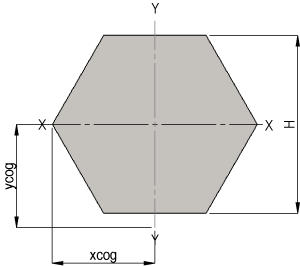SECTIONAL PROPERTIES CALCULATOR - SOLID HEXAGON

Enter your values and select the units as required.PARAMETERSYMBOLVALUEUNITS
HEIGHTH
LENGTHL
DENSITYp
RESULTS
CROSS SECTION AREAA
MASSM
SECOND MOMENT OF AREA (lxx)lxx
SECOND MOMENT OF AREA (lyy)lyy
SECTION MODULUS (Sxx)Sxx
SECTION MODULUS (Syy)Syy
RADIUS OF GYRATION (rx)rx
RADIUS OF GYRATION (ry)ry
CoG DISTANCE IN X DIRECTION (xcog)xcog
CoG DISTANCE IN Y DIRECTION (ycog)ycog

Definitions:

Radius of Gyration (Area): The capacity of a cross-section to resist bending.

Second Moment of Area: The distance from an axis at which the area of a body may be assumed to be concentrated and the second moment area of this configuration equal to the second moment area of the actual body about the same axis.

Section Modulus: The moment of inertia of the area of the cross section of a structural member divided by the distance from the center of gravity to the farthest point of the section; a measure of the flexural strength of the beam.